• 例如：一个综合性的excel报表，第1行C列是省份，而且此综合性报表有多个sheet要拆分，想要实现拆分所有sheet，并按省份合并成一个excel表，比如所有辽宁省的数据合并成一个excel文件以不同的sheet体现。用此脚本即可...
• 拆分表格并保存函数Application主程序对象Open 打开文件Add 新建工作簿练习拆分表存储成文件利用筛选分离表格内容 函数 Application主程序对象 application.displayalsert=false 表示不要弹窗 Open 打开文件 eg ： ...

函数

Application主程序对象

application.displayalsert=false 表示不要弹窗

Open 打开文件

eg ：

application screenupdating=false//不要屏幕更新
Workbooks. Open Filename:="d:\data\1.xlsx"
activeworkbook.sheets(1).range("a1") = "lala"//表示打开当前文件输入的标记
activeworkbook.save
activeworkbook.close
application.displayalsert=true

eg:

Sub one()
ActiveWorkbook.Sheets(1).Range("a1") = "llll"
ActiveWorkbook.SaveAs Filename:="C:\Users\zsnzd\Desktop\excel\第四节\22.xlsx"
ActiveWorkbook.Close

End Sub

range（“范围”）
**Save/Save as 保存工作簿
Close关闭工作簿
Select(选中)
Delete(删除)
Copy(复制)
ClearContents(清空)
Value(值) Text(内容文字)
Row(行号) Column(列号)
Entirerow单元格所在整行 **

新建表时回避重名错误

Msgbox和Inputbox窗口函数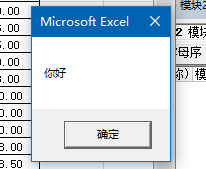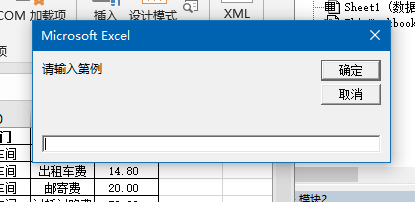Sub chuan()
Msgbox "你好"
m = InputBox("请输入第" & m & "例")
End Sub

练习

拆分表存储成文件

注意i容易出错

Sub chaifen()
Dim sht As Worksheet
Dim i As Integer
For Each sht In Sheets

sht.Copy
ActiveWorkbook.SaveAs Filename:="C:\Users\zsnzd\Desktop\excel\第四节\" & sht.Name & ".xlsx"
ActiveWorkbook.Close

Next
End Sub

结合上一讲综合练习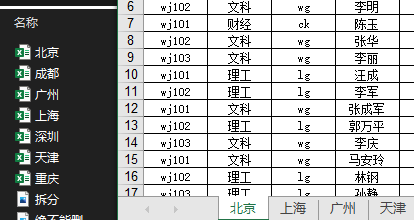//删除空格，填充
Sub shaixuan()
Dim sht As Worksheet
Dim i As Integer

For Each sht In Sheets
For i = 100 To 2 Step -1
If sht.Cells(i, 4) = "" Then
sht.Range("d" & i).EntireRow.Delete
End If

If sht.Cells(i, 2) = "理工" Then
sht.Cells(i, 3) = "lg"
ElseIf sht.Cells(i, 2) = "文科" Then
sht.Cells(i, 3) = "wg"
Else
sht.Cells(i, 3) = "ck"
End If

If sht.Cells(i, 5) = "男" Then
sht.Cells(i, 6) = "先生"
Else
sht.Cells(i, 6) = "女士"
End If
Next

Next

End Sub

//拆成文件
Sub chai()
Dim sht As Worksheet
For Each sht In Sheets
sht.Copy
ActiveWorkbook.SaveAs Filename:="C:\Users\zsnzd\Desktop\excel\第四节\" & sht.Name & ".xlsx"
ActiveWorkbook.Close
Next
End Sub

利用筛选分离表格内容

**注意
1、end后面的row
2、k是整数型
3、range（“a1：f”&k）
4、copy后面直接加sht.单元格
5、Criteria1:="=" 这里是one
**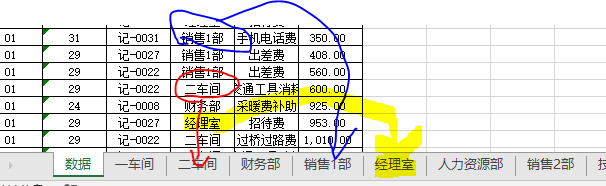Sub 用筛选拆分()
Dim i As Integer
Dim sht As Worksheet

i = Sheet1.Range("a65535").End(xlUp).Row
For Each sht In Worksheets
If sht.Name <> 数据 Then

Sheet1.Range("a1:f" & i).AutoFilter field:=4, Criteria1:="=" & sht.Name
Sheet1.Range("a1:f" & i).Copy sht.Range("a1")

End If
Next

Sheet1.Range("a1:f" & i).AutoFilter
End Sub

按照a1建表（避免重复名字）

**注意：
1、i表示对行的循环整数
2、k表示布尔值判断作用
**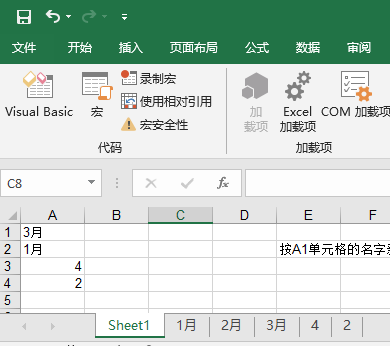Sub bimian()
Dim sht As Worksheet
Dim i As Integer
Dim k As Integer
For i = 1 To Sheet1.Range("a65536").End(xlUp).Row
k = 0
For Each sht In Sheets
If sht.Name = Sheet1.Range("a" & i) Then
k = 1
End If
Next
If k = 0 Then
Sheets(Sheets.Count).Name = Sheet1.Range("a" & i)
End If
Next
End Sub

填充数据到指定表格里

Sub chai()
Dim sht As Worksheet
Dim k As Integer
k = Sheet1.Range("a65536").End(xlUp).Row
For Each sht In Sheets
If sht.Name <> 数据 Then
Sheet1.Range("a1:f" & k).AutoFilter field:=4, Criteria1:="=" & sht.Name
Sheet1.Range("a1:f" & k).Copy sht.Range("a1")
Next
Sheet1.Range("a1:f" & k).AutoFilter
End Sub

将数据表单元格创建分表再填充数据

Sub chai()
Dim sht As Worksheet
Dim k, i, j As Integer
k = Sheet1.Range("a65536").End(xlUp).Row
'拆分
For i = 2 To k
j = 0
For Each sht In Worksheets
If sht.Name = Sheet1.Range("d" & i) Then
j = 1
End If
Next
If j = 0 Then
Sheets(Sheets.Count).Name = Sheet1.Range("d" & i)
End If
Next
'填数据
For Each sht In Worksheets
If sht.Name <> 数据 Then
Sheet1.Range("a1:f" & k).AutoFilter field:=4, Criteria1:="=" & sht.Name
Sheet1.Range("a1:f" & k).Copy sht.Range("a1")
End If
Next

Sheet1.Range("a1:f" & k).AutoFilter
End Sub

综合训练

利用窗口函数吧上面的动作重新来一遍

Sub chai()
Dim sht As Worksheet
Dim k, i, j As Integer
k = Sheet1.Range("a65536").End(xlUp).Row
Msgbox "你好"
m = InputBox("请输入一个" & m & "列")
'拆分
For i = 2 To k
j = 0
For Each sht In Worksheets
If sht.Name = Sheet1.Range("d" & i) Then
j = 1
End If
Next
If j = 0 Then
Sheets(Sheets.Count).Name = Sheet1.Range("d" & i)
End If
Next
'填数据
For Each sht In Worksheets
If sht.Name <> 数据 Then
Sheet1.Range("a1:f" & k).AutoFilter field:=m, Criteria1:="=" & sht.Name
Sheet1.Range("a1:f" & k).Copy sht.Range("a1")
End If
Next

Sheet1.Range("a1:f" & k).AutoFilter
End Sub

把分表内容整合到sht1

注意
1、清空内容要指定range范围

Sub hebing()
Dim i, j As Integer   //i是数据源表的最后一行，j是目标表(数据表)的最后一行
Dim sht As Worksheet

//先要删除所有数据
Sheet1.Range("a1:f65536").ClearContents

//复制表头
Sheet2.Range("a1:f1").Copy Sheet1.Range("a1")

//复制数据
For Each sht In Sheets
If sht.Name <> "数据" Then
i = sht.Range("a65536").End(xlUp).Row
j = Sheet1.Range("a65536").End(xlUp).Row

sht.Range("a2:f" & i).Copy Sheet1.Range("a" & j + 1)
End If
Next
End Sub

展开全文excel vba csv
• 1、将总表根据【销售部门】拆分成不同的表格 2、拆分后保持格式不变 拆分前 总表 拆分后 表结构 一部 二部 七部 代码如下 Sub cfgzb() '拆分工作表 Dim i As Integer, endrow As Integer, irow As ...

需求

1、将总表根据【销售部门】拆分成不同的表格

2、拆分后保持格式不变

代码如下

Sub cfgzb() '拆分工作表
Dim i As Integer, endrow As Integer, irow As Integer
Dim sh As Worksheet
Dim str As String
endrow = Sheets("总表").Range("c" & Rows.Count).End(xlUp).Row '找到最后一行的万金油公式
For i = 3 To endrow
str = Sheets("总表").Range("c" & i).Value '把部门名称放入字符串str中
On Error Resume Next '从该语句开始，遇到错误程序不会中止，也不会出现错误提示，将继续运行
Set sh = Sheets(str) 'sh是工作表
If Err.Number = 0 Then '如果部门表存在
irow = sh.Range("a" & Rows.Count).End(xlUp).Row + 1 '保证新复制的数据不会覆盖原有的
Sheets("总表").Rows(i).Copy sh.Rows(irow)
Else '如果部门表不存在
sh.Name = str '重命名
sh.Move , Sheets(Sheets.Count) '移动工作表
Sheets("总表").Rows(1).Copy sh.Rows(1) '按行复制，保留行高
Sheets("总表").Rows(2).Copy sh.Rows(2)
Sheets("总表").Rows(i).Copy sh.Rows(3)
With sh.Cells(3, "a").Resize(1, 8)
.PasteSpecial xlPasteFormats  '选择性粘贴格式
.PasteSpecial xlPasteColumnWidths '选择性粘贴列宽
End With
End If
On Error GoTo 0
Next i
MsgBox "拆分完成" '全部完成会有一个提示语句
End Sub

更新版本

需求

在第一版的基础上表头出现纵向合并

代码如下

Sub cfgzb() '拆分工作表
Dim i As Integer, endrow As Integer, irow As Integer
Dim sh As Worksheet
Dim str As String
endrow = Sheets("总表").Range("c" & Rows.Count).End(xlUp).Row '找到最后一行的万金油公式
For i = 5 To endrow
str = Sheets("总表").Range("c" & i).Value '把部门名称放入字符串str中
On Error Resume Next '从该语句开始，遇到错误程序不会中止，也不会出现错误提示，将继续运行
Set sh = Sheets(str) 'sh是工作表
If Err.Number = 0 Then '如果部门表存在
irow = sh.Range("a" & Rows.Count).End(xlUp).Row + 1 '保证新复制的数据不会覆盖原有的
Sheets("总表").Rows(i).Copy sh.Rows(irow)
Else '如果部门表不存在
sh.Name = str '重命名
sh.Move , Sheets(Sheets.Count) '移动工作表
Sheets("总表").Range("A1:H3").Copy sh.Range("A1:H3")
Sheets("总表").Rows(4).Copy sh.Rows(4) '按行复制，保留行高
Sheets("总表").Rows(i).Copy sh.Rows(5)
With sh.Cells(5, "a").Resize(1, 8)
.PasteSpecial xlPasteFormats  '选择性粘贴格式
.PasteSpecial xlPasteColumnWidths '选择性粘贴列宽
End With
End If
On Error GoTo 0
Next i
MsgBox "拆分完成" '全部完成会有一个提示语句
End Sub

展开全文• 背景：业务给了一个大表格，里面几十万条数据，要拆分成成百上千个小表格，思来想去，vba做这件事是效率最高的。 样表数据源： 请按照这个表头在excel中制作样表(最好将样表放在一个空文件夹里面) 然后调出VB编辑器...

背景：业务给了一个大表格，里面几十万条数据，要拆分成成百上千个小表格，思来想去，vba做这件事是效率最高的。

样表数据源：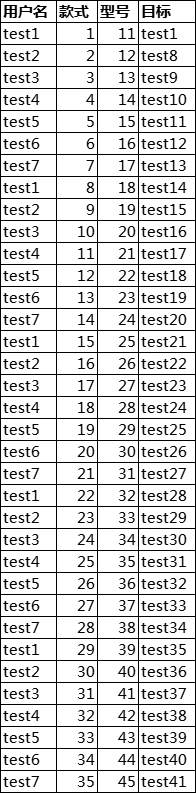请按照这个表头在excel中制作样表(最好将样表放在一个空文件夹里面)
然后调出VB编辑器，输入如下代码运行

Sub 按A列区分内容并拆分到新表格()

Dim i%

arr = Sheets(1).[a1].CurrentRegion

Set d = CreateObject("scripting.dictionary")

For i = 2 To UBound(arr)

If d.exists(arr(i, 1)) Then  '判断key是否存在

Set d(arr(i, 1)) = Union(d(arr(i, 1)), Rows(i))  '如果存在则将前面相同key的行和当前key对应的行合并成一个对象

Else

Set d(arr(i, 1)) = Union(Rows(1), Rows(i))   '如果不存在则把表头拿过来和当前行合并成一个对象

End If

Next i

For ss = 0 To d.Count - 1

With ActiveWorkbook

d.items()(ss).Copy .Sheets(1).[a1]   '将列A每一个值对应的行单独拿出来，粘贴复制到一个新表格

.SaveAs ThisWorkbook.Path & "/" & d.keys()(ss)  '每个新表格的名字是列A的每一个值

.Close

End With

Next ss

MsgBox "工作薄拆分完毕！"

End Sub

运行结果如下：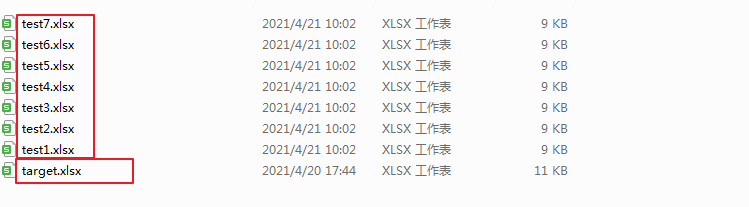一个表格拆成7个小表格

补充第二个情景：
按照每10行做为一个表格，切分成多个表格

Sub 按A列区分内容并拆分到新表格()

Dim i%
arr = Sheets(1).[a1].CurrentRegion
For i = 2 To UBound(arr)
If i Mod 10 = 0 Then
With ActiveWorkbook
Union(Workbooks("test.xlsx").Sheets(1).Range("a1:e1"), Workbooks("test.xlsx").Sheets(1).Range("a" & (i - 9) & ":e" & i)).Copy .Sheets(1).[a1]
.SaveAs ThisWorkbook.Path & "/" & i

.Close

End With
End If

Next i

MsgBox "工作薄拆分完毕！"

End Sub

展开全文vba excel
• 2、excel表格保留一行标题行，并把第一列数据填写为拆分项（文件拆分时将按第一列的内容进行归类合并为一个文件），整个表格不要合并单元格； 3、在打开的EXCEL工作表名称上点右键选择查看代码； 4、选择菜单栏：...
• VBA代码拆分excel

千次阅读 2017-08-12 00:22:20
忙活两小时，终于帮老姐实现了拆分工作excel的需求，记录下，说不定以后可以用到。。 需求：一个excel文件工作簿可能包含多个工作表(比如sheetA，sheetB,sheetC),每个sheet里每一行都有一个地市字段，现需要根据地市...

忙活两小时，终于帮老姐实现了拆分工作excel的需求，记录下，说不定以后可以用到。。          需求：一个excel文件工作簿可能包含多个工作表(比如sheetA，sheetB,sheetC),每个sheet里每一行都有一个地市字段，现需要根据地市拆分成不同的excel（每个excel包含sheetA，sheetB,sheetC，而且每个sheet里的记录都是同一个地市的）。                                               代码如下：                    Sub 复制表()                              Dim j%                                For j = 1 To 21                                 ThisWorkbook.SaveCopyAs ThisWorkbook.Path & "\" & Split(ThisWorkbook.Name, ".xlsm")(0) & j & ".xlsm"                              Next                          Call 打开并关闭文件                          End Sub                          Sub 打开并关闭文件()                          Dim wb As Workbook                          Dim m                                                     For m = 1 To 21                              Set wb = Workbooks.Open(ThisWorkbook.Path & "\" & Split(ThisWorkbook.Name, ".xlsm")(0) & m & ".xlsm", True)                                                                                Call 拆分(m)                                                     Sheets("sheet1").Visible = xlSheetVeryHidden                                                     wb.Close True                                                     Next                                                     End Sub                          Sub 拆分(m)                          Dim B1                          Dim k%                          k = m                              Sheets("KPI").Select                              For B1 = 24 To 3 Step -1                                  If Cells(B1, 1) <> Sheets("sheet1").Range("A" & k).Value Then                                      Rows(B1 & ":" & B1).Select                                      Selection.Delete Shift:=xlUp                                  End If                              Next B1                                                            Sheets("大规模断站8月").Select                              For B1 = 24 To 3 Step -1                                  If Cells(B1, 1) <> Sheets("sheet1").Range("A" & k).Value Then                                      Rows(B1 & ":" & B1).Select                                      Selection.Delete Shift:=xlUp                                  End If                              Next B1                                                            Sheets("大规模断站明细").Select                              B1 = Range("A1").End(xlDown).Row 'b1等于a列有数据最后一行的行号                              While Cells(B1, 5) <> "分公司"                                  If Cells(B1, 5) <> Sheets("sheet1").Range("A" & k).Value Then                                      Rows(B1 & ":" & B1).Select                                      Selection.Delete Shift:=xlUp                                  End If                              B1 = B1 - 1                           Wend                          End Sub                                                     PS：上述代码需要根据实际情况调整！！
展开全文拆分excel
• 所以针对这个问题，用VBA写了个分列和保存的宏。 一、使用前说明： 1.要分列的表列中间不要出现空内容，比如要分第2列部门，里面有A部门，B部门，还有个空内容的，可能导致计算行数不准。 2.分列后的表序号...
• 如图，这边需要按照工作内容拆分多个工作表，直接上代码： Sub splitSht() Dim sht As Worksheet Dim d As Object Dim j As Integer Set sht = ThisWorkbook.Worksheets("Sheet1") Set d = CreateObject(...vba
• 一、需要将工作簿里面不同的sheet快速拆分成为不同的工作簿 二、代码 Sub 分拆工作表() Dim shAs Worksheet Dim MyBook As Workbook Set MyBook = ActiveWorkbook For Each shIn MyBook.Sheets sh.Copy...
• Sub 表格关键词拆分() Application.ScreenUpdating = False Dim x As Integer, y As Integer, w As Worksheet inputcol = InputBox("请输入拆分列所在序号") x = 2 y = inputcol Set w = Worksheets(1) Do While w....vba excel
• 将总数据表进行拆分至多个工作表问题使用For循环拆分工作表使用筛选拆分工作表结果图 问题 将数据表中数据根据所属部门分配至对应部门的工作表内，如下图所示： 使用For循环拆分工作表 Sub shishi() Dim sht As ...excel vba
• 使用情景：如题，将一个包含多工作表的工作簿拆分为单工作表工作簿文件 原始文件描述：【旺旺大礼包.xlsx】中有三个工作表，分别是旺仔牛奶、旺旺仙贝、旺旺小小酥 操作流程： 打开旺旺大礼包.xlsx——在菜单栏...
• Sub DetachWorkbook() On Error Resume Next Dim pathStr As String, i As Long, activeWb As String With Application.FileDialog(msoFileDialogFolderPicker) If .Show Then pat...
• VBA拆分excel

2015-08-06 14:02:35
VBA拆分excel表格，最快的分表，详情请下载观看。VBA
• 即打开VBA的模块粘贴下面代码后按f5运行,会在该目录下得到拆分后的文件.Sub test()Application.ScreenUpdating = Falsep = ThisWorkbook.Path &amp; "\"With ActiveSheetFor r = 1 To .Range("a...
• 实用VBA工具，一步步摆脱工具人 把我给的代码，复制到VBA里面，直接运行，就ok 亲测有效。 有问题请留言~ Sub 保留表头拆分数据为若干新工作簿() Dim arr, d As Object, k, t, i&, lc%, rng As Range, c% c =...
• VBA代码实例---一个工作表拆分为N个工作表

万次阅读 多人点赞 2017-04-18 22:53:29
这是一个常用而且经典的例子：根据内容，把一个工作表中的内容，拆分到N个工作表中，并根据内容命名新建的工作表。 ¤主要知识点¤ 1、影响代码执行闪屏以及提示框的处理： Application.ScreenUpdating = False ...
• 有时候时候需要将一个大的excel表格拆分成多个文件，这里通过VBA提供一种方法 步骤一 打开VB编辑器 步骤二 插入模块 步骤三 复制代码到模块中 步骤四 运行代码 Sub 保留表头拆分以表头命名新工作簿() Dim arr,...
• Sub 保留表头拆分数据为若干新工作簿() Dim arr, d As Object, k, t, i&, lc%, rng As Range, c% c = Application.InputBox("请输入拆分列号", , 4, , , , , 1) If c = 0 Then Exit Sub Application....
• VBA–同一个工作簿中如何拆分工作表 Sub 拆分工作表() Dim sht As Worksheet Dim wb As Workbook For Each sht In Worksheets sht.Copy Set wb = ActiveWorkbook wb.SaveAs Filename:=thisworkbook.path & “” ...vba
• VBA -- 实现按指定条件拆分工作表的功能

万次阅读 多人点赞 2018-05-16 22:38:26
本文介绍了通过VBA拆分工作表的方法，也抛出了个人公众号的“VBA引子”。VBA语言比较简单易懂，而且对实际工作有很大的帮助，后期文章将根据情况介绍VBA基础及其他高阶的应用。另外下一个“引子”打算抛出python爬虫...VBA 拆分表格 工作效率 数据 excel
• Excel VBA 宏 拆分Excel表

千次阅读 2017-02-08 13:28:51
问题： excel 文件 如下： num char key 1 a 关键字1 1 a 关键字1 1 a 关键字1 1 a 关键字1 2 b 关键字2 2 b 关键字2 2 b 关键字2 2 b 关键字2 ...按照关键字拆分成 多个 excle 表（非she
• Word使用VBA实现拆分文档页数实现步骤： 新建一个记事本，添加以下代码 Sub 利用VBA实现Word文档的拆分() On Error Resume Next Dim doc1 As Document, newdoc As Document, a, page1%, page2%, i1%, i2%, i3%, dk...程序人生 经验分享 websocket http
• 这里需要按城市（即B列数据）对表格进行拆分拆分出多个以城市名称命名的xlsx文件，每个xlsx文件都只包含当前城市的数据。  相关资料 之前没有接触过Excel相关的编程，也没有学习过VB语言，完全是摸着...excel vba 拆分工作表
• 当单元格进行合并之后就是如何进行拆分拆分和合并原理大致相似，就是先判断是否是合并单元格，然后获取合并单元格的数目，进行拆分和填充。相应代码如下： Sub 拆分单元格() Dim i, l, m As Integer '禁止弹...
• 多种方式拆分EXCEL文件。1、将表拆分至不同的文件；2、将同一张表中数据按内容不同拆分至不同的表或者文件；3、将同一张表中数据按指定行数拆分至不同的表或者文件。EXCEL 拆分
• 有这样一个表,想用筛选的方式,减少for循环,让D列的相同名称的数据复制到后面对应的工作表里 代码如下: Sub shaifen() Dim i As Integer For i = 2 To Sheets.Count Sheet1.Range(“a1:f1048”).AutoFilter Field:=4...
• 首先，将需要拆分的sheet命名为“明细”，接下来运行此代码，按提示操作即可。 在这里插入代码片 Sub chaifen() '定义变量类型 Dim sht, sh1, sh2 As Worksheet Dim k, i, j As Integer Dim irow As Integer Dim col...Excel VBA
• 可以看到，该Excel表格的A列每一行都有两行的数据，而我们的目的就是把这七行进行拆分为十四行，每一行包含单元格内单行的一条信息，其余列的文件进行复制，如下图所示。 解决方案 在这里提供一个可设定的解决方案...
• Public Sub 多个区域拆分到多表() AppSettings On Error GoTo ErrHandler Dim StartTime, UsedTime As Variant StartTime = VBA.Timer 'Input code here Dim Wb As Workbook Dim sht As......